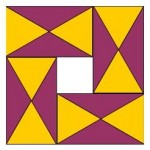If you take the edge length of the equilateral triangles in this picture as the unit for length, what would be the exact size of the hole ?

Alternatively, if the area of an equilateral triangles is taken as the unit for area, what size is the hole?